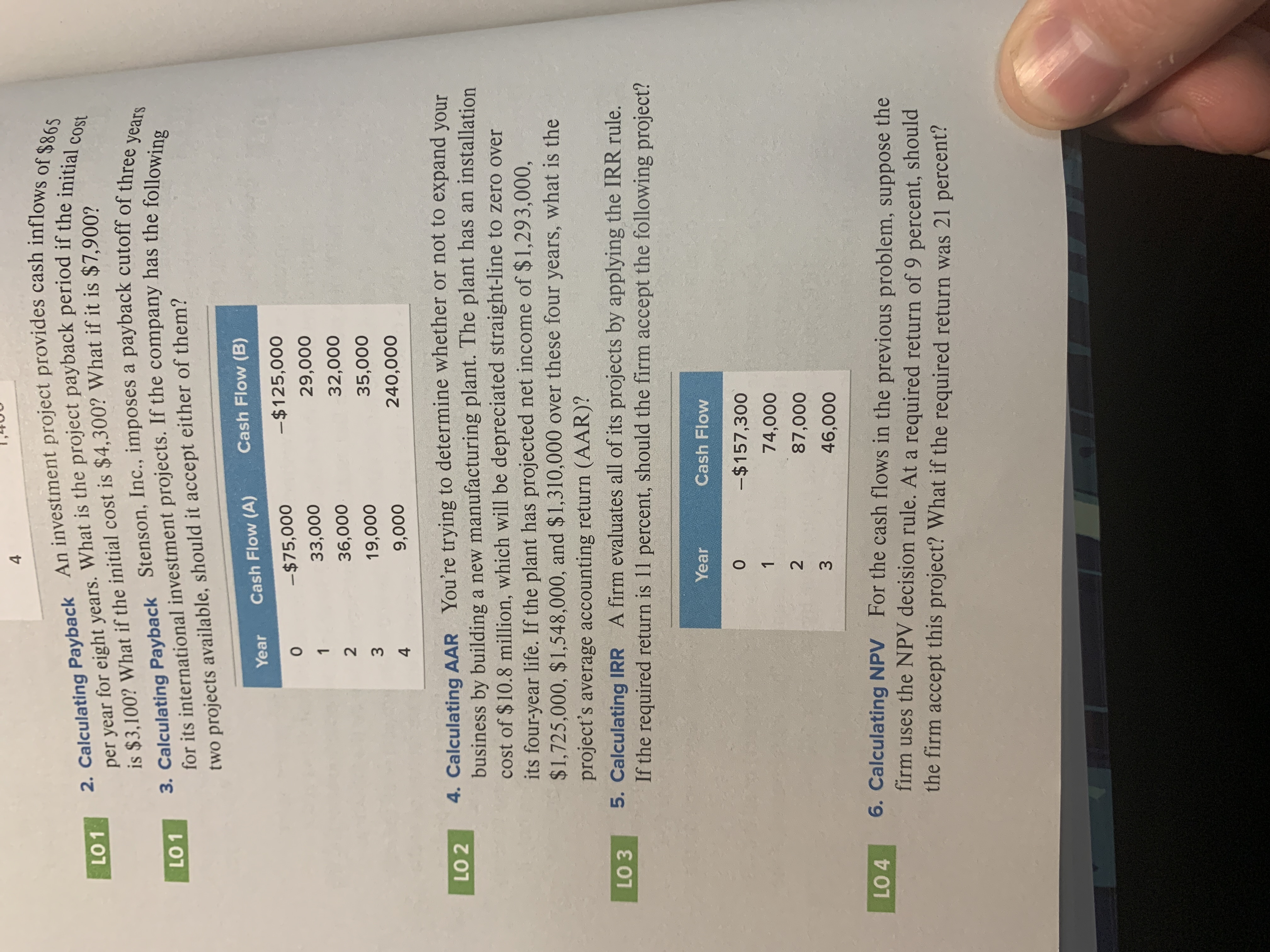# 42. Calculating Payback An investment project provides cash inflows of \$865per year for eight years. What is the project payback period if the initial costis \$3,100? What if the initial cost is \$4,300? What if it is \$7.900?3. Calculating Payback Stenson, Inc., imposes a payback cutoff of three yearsfor its international investment projects. If the company has the followingLO 1LO 1two projects available, should it accept either of them?Cash Flow (B)Cash Flow (A)Year-\$125,000-\$75,00029,00033,000132,00036,00035,00019,0003240,0009,0004. Calculating AAR You're trying to determine whether or not to expand yourbusiness by building a new manufacturing plant. The plant has an installationcost of \$10.8 million, which will be depreciated straight-line to zero overits four-year life. If the plant has projected net income of \$1,293,000,\$1,725,000, \$1,548,000, and \$1,310,000 over these four years, what is theproject's average accounting return (AAR)?LO 25. Calculating IRR A firm evaluates all of its projects by applying the IRR rule.If the required return is 11 percent, should the firm accept the following project?LO 3YearCash Flow-\$157,300174,000287,000346,0006. Calculating NPV For the cash flows in the previous problem, suppose thefirm uses the NPV decision rule. At a required return of 9 percent, shouldthe firm accept this project? What if the required return was 21 percent?LO 4

Question
129 views

Number 5 and 6help_outlineImage Transcriptionclose4 2. Calculating Payback An investment project provides cash inflows of \$865 per year for eight years. What is the project payback period if the initial cost is \$3,100? What if the initial cost is \$4,300? What if it is \$7.900? 3. Calculating Payback Stenson, Inc., imposes a payback cutoff of three years for its international investment projects. If the company has the following LO 1 LO 1 two projects available, should it accept either of them? Cash Flow (B) Cash Flow (A) Year -\$125,000 -\$75,000 29,000 33,000 1 32,000 36,000 35,000 19,000 3 240,000 9,000 4. Calculating AAR You're trying to determine whether or not to expand your business by building a new manufacturing plant. The plant has an installation cost of \$10.8 million, which will be depreciated straight-line to zero over its four-year life. If the plant has projected net income of \$1,293,000, \$1,725,000, \$1,548,000, and \$1,310,000 over these four years, what is the project's average accounting return (AAR)? LO 2 5. Calculating IRR A firm evaluates all of its projects by applying the IRR rule. If the required return is 11 percent, should the firm accept the following project? LO 3 Year Cash Flow -\$157,300 1 74,000 2 87,000 3 46,000 6. Calculating NPV For the cash flows in the previous problem, suppose the firm uses the NPV decision rule. At a required return of 9 percent, should the firm accept this project? What if the required return was 21 percent? LO 4 fullscreen
check_circle

Step 1

5) The IRR is the rate where NPV equals to zero.

Using the goal seek method, IRR is identified as 16.26%, which is greater than the required return of 11%, thus the project must be accepted.

Step 2

6. At a return of 9%, NPV is as follows:

The net present value is positive, thus the project mus...

### Want to see the full answer?

See Solution

#### Want to see this answer and more?

Solutions are written by subject experts who are available 24/7. Questions are typically answered within 1 hour.*

See Solution
*Response times may vary by subject and question.
Tagged in